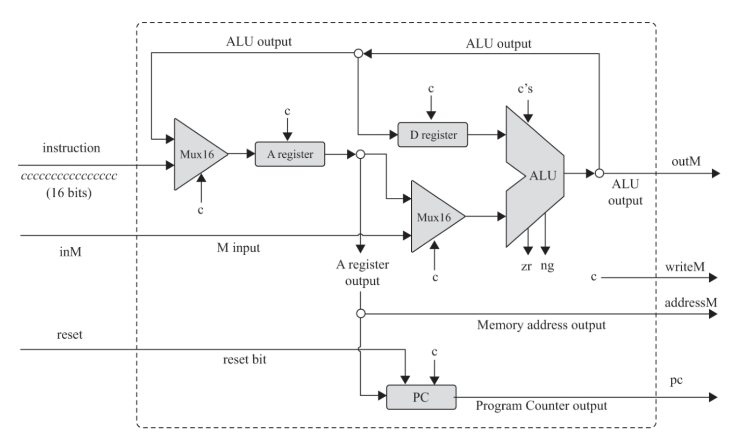# Why we need ALU "feedback" in the registers? (CPU implementation)ClassicListThreaded6 messagesOpen this post in threaded view
|

## Why we need ALU "feedback" in the registers? (CPU implementation)

 I don't understand the logic behind ALU "feedback" in A and D registers. why do we need it?
Open this post in threaded view
|

## Re: Why we need ALU "feedback" in the registers? (CPU implementation)

 I'm not sure what you mean by "feedback" here. If you mean why we connect the ALU output to the A or D registers, then the answer is, that we need to do that in order to implement assembly instructions like A=A+D or D=D+1.
Open this post in threaded view
|

## Re: Why we need ALU "feedback" in the registers? (CPU implementation)

 yes I mean just that. can you explain how we are doing A=A+D or D=D+1 ? my logic is: at time t=0, C instruction arrives. Since this is a C instruction, the mux selects the previous ALU output which can be anything. And since load bit of the C instruction is 1, the A register is loaded with the previous ALU value. This value may not be the same as what we want from our C instruction (in this case, A+D). this is where I am confused.
Open this post in threaded view
|

## Re: Why we need ALU "feedback" in the registers? (CPU implementation)

 The registers are implemented using data flip-flops (DFFs), so you need to understand how that works. The DFF, as implemented in this course, can remember a single bit for a single time-cycle. What does this mean? First of all, time is measured by the clock signal. You can think of it as a wire, which connects to all DFFs in the computer and which changes from 0 to 1 to 0 to 1 to 0 to 1 and so forth at regular rate. In the simulator, this wire is not explicitly shown, it's just that every DFF you create is connected to it. And you progress the time by pressing the tick/tock button. Basically, tick means that the clock goes from 0 to 1, and tock means that it goes from 1 to 0. Now the DFF remembers its input when the clock goes from 1 to 0. This means that whatever happens to its input at any other time, the output will not be affected. This is how the cycle is "broken" and the register can both be an input to the ALU and the place to store the value of the ALU computation. Please note, that I don't remember the exact details from the book. You'll have to check if the tick and tock are actually these transitions or the reversed ones and if the DFF is remembering its input when the clock goes from 1 to 0 or from 0 to 1. These are all details of the implementation, but the principle is the same in both cases.
 sorry if I missed your point but I do know these details. But I still don't understand how A=A+D or D=D+M etc. commands are running in this CPU implementation. here is the implementation of the CPU if you need it: